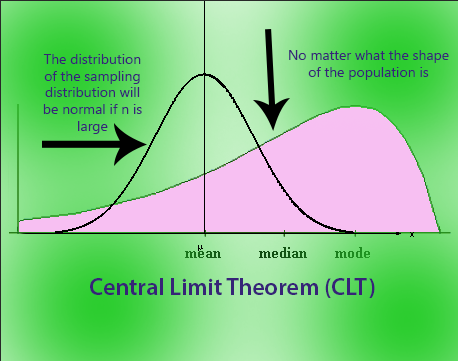Bean Machinemore_vert
Bean Machineclose

This is a Bean Machine which simulates the Central Limit Theorem. It shows that with a large sample size, a binomial distribution approximates a normal distribution.Pendulummore_vert
Pendulumclose

This is a Pendulum which simulates oscillatory motion. In particular, this motion is simple harmonic motion because the force is proportional to the displacement of the pendulum and it can be modeled by sinusoidal functions (sin, cos).Parametric Grapherclose

This is a simulation it simulates and is very educational.

Calculatormore_vert
Calculatorclose

This is a simple 4 function calculator which uses the basic operators that we have all learned in elementary school.

Simulationmore_vert
Simulationclose

This is a simulation it simulates and is very educational.

Simulationmore_vert
Simulationclose

This is a simulation it simulates and is very educational.